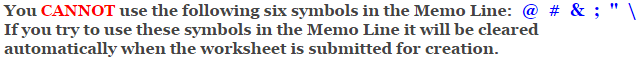# Calculus

## Second Fundamental Theorem of Calculus Worksheets

These Calculus Worksheets will produce problems that involve using the second fundamental theorem of calculus to find derivatives.
The student will be given an integral of a polynomial function and will be asked to find the derivative of the function.
You may select the number of problems, and the types of functions.

These Second Fundamental Theorem of Calculus Worksheets are a great resource for Definite Integration.

### Language for the Second Fundamental Theorem of Calculus Worksheets

 English German Albanian Spanish Swedish Italian French Turkish Polish Norwegian

### Memo Line for the Second Fundamental Theorem of Calculus Worksheets

You may enter a message or special instruction that will appear on the bottom left corner of the Second Fundamental Theorem of Calculus Worksheets.### Second Fundamental Theorem of Calculus Worksheets Answer Page

Now you are ready to create your Second Fundamental Theorem of Calculus Worksheets by pressing the Create Button.

 Recommended Videos

If You Experience Display Problems with Your Math Worksheet